# Equations Inequalities Fraction Equations Geometry Graphing X Y

• Slides: 30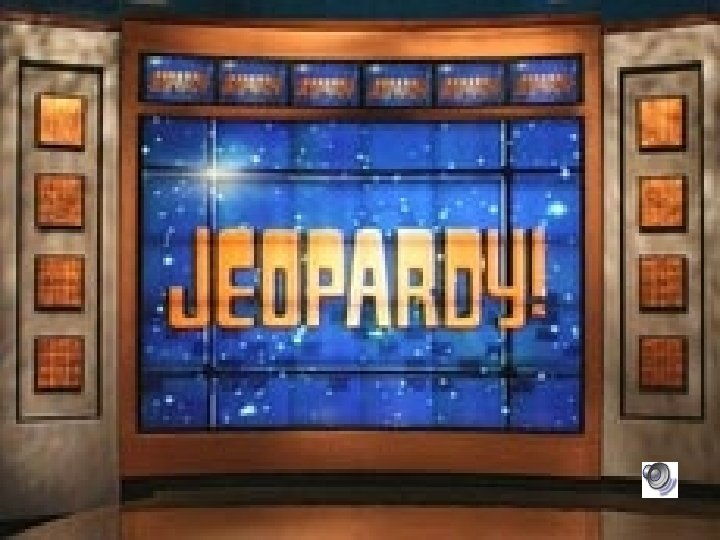Equations Inequalities Fraction Equations Geometry Graphing (X, Y) 100 100 100 200 200 200 300 300 300 400 400 400 500 500 500 Final JeopardySolve and check the following equation: T + 27 = 54 100 2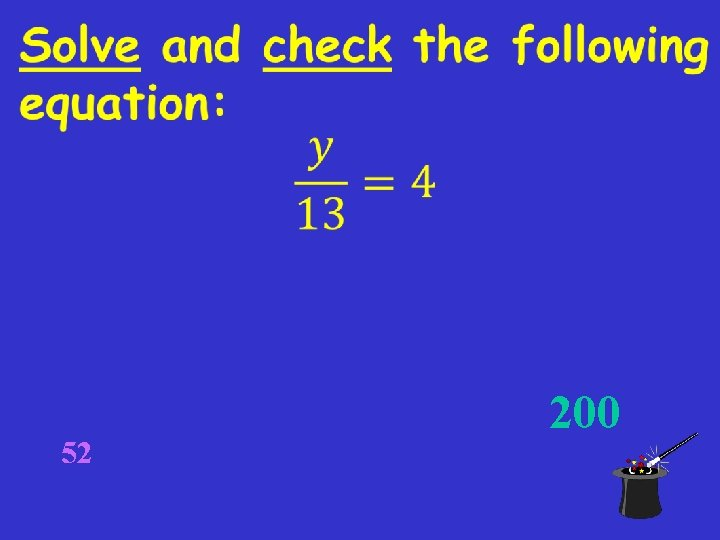52 200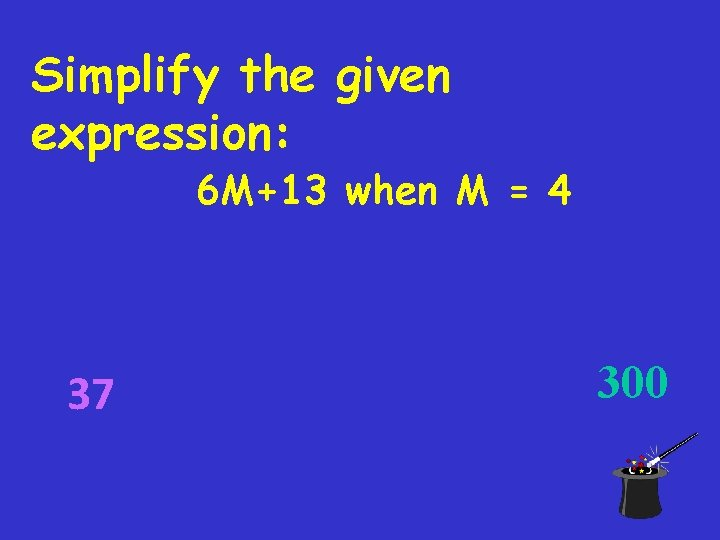Simplify the given expression: 6 M+13 when M = 4 37 300Simplify the given expression: 7 G+3 G-4 G+10 when G= 2 22 400To solve we need to get A alone we need to use inverse operations, so we multiply by six on both sides. 20 times 6 is 120. so A equals 120 500Graph the following inequality: R ≤ 18 Teacher drawn 100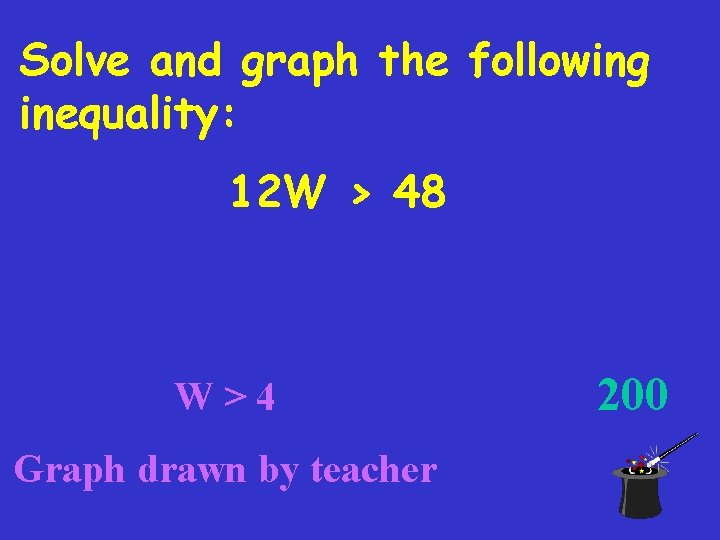Solve and graph the following inequality: 12 W > 48 W>4 Graph drawn by teacher 200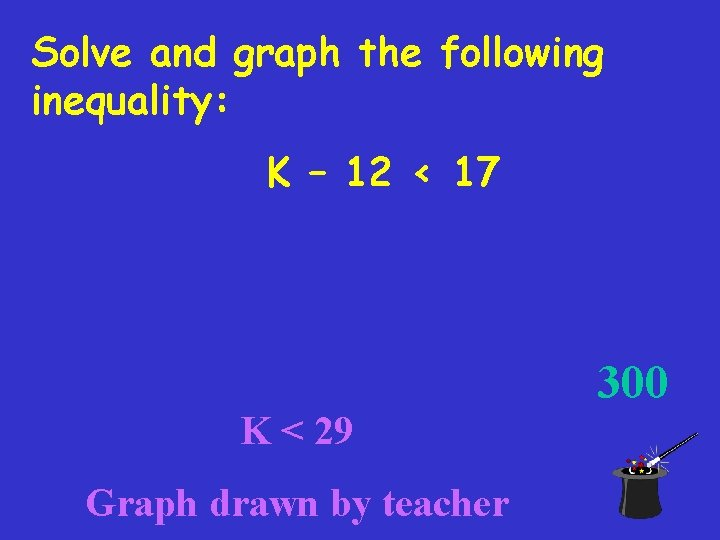Solve and graph the following inequality: K – 12 < 17 300 K < 29 Graph drawn by teacher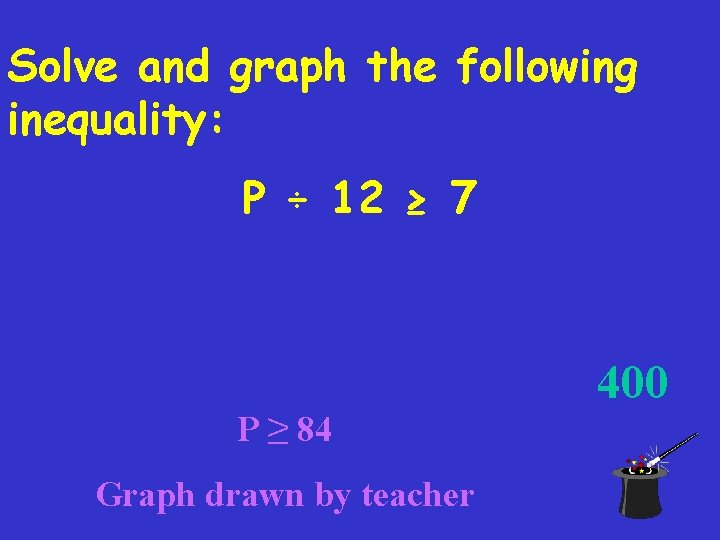Solve and graph the following inequality: P ÷ 12 ≥ 7 400 P ≥ 84 Graph drawn by teacher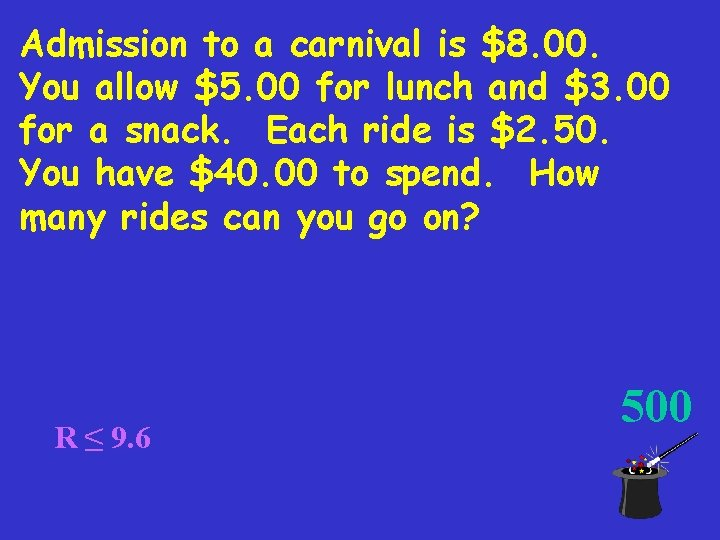Admission to a carnival is \$8. 00. You allow \$5. 00 for lunch and \$3. 00 for a snack. Each ride is \$2. 50. You have \$40. 00 to spend. How many rides can you go on? R ≤ 9. 6 500What do we need when we add and subtract fractions? Common denominators 100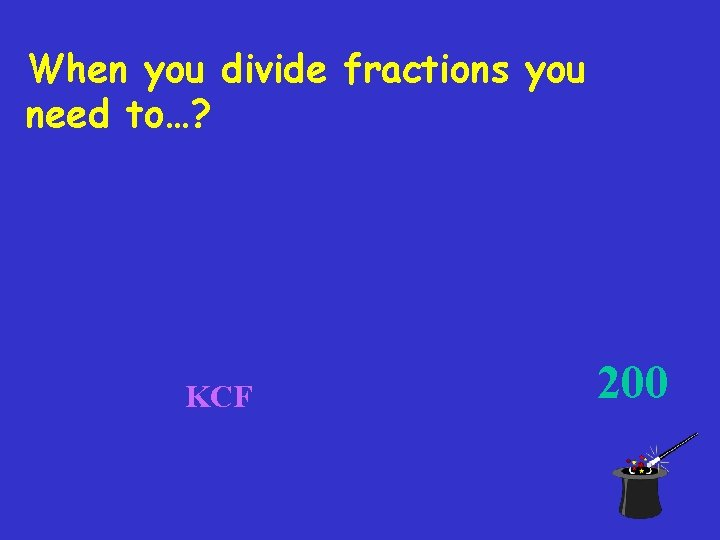When you divide fractions you need to…? KCF 200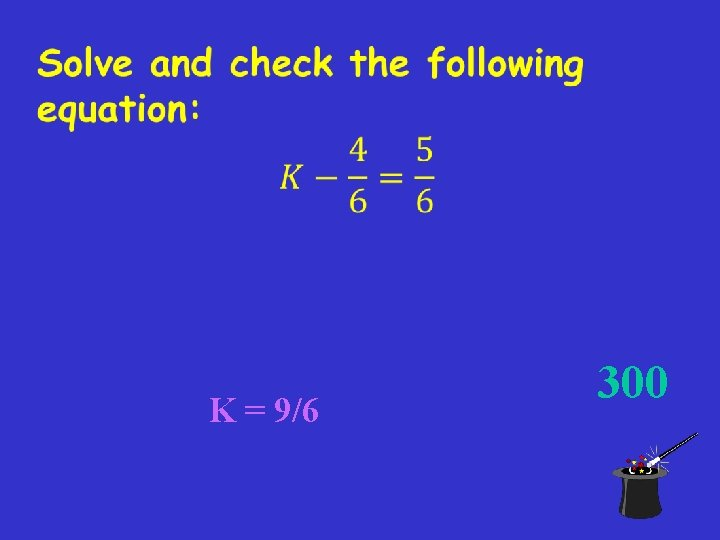K = 9/6 300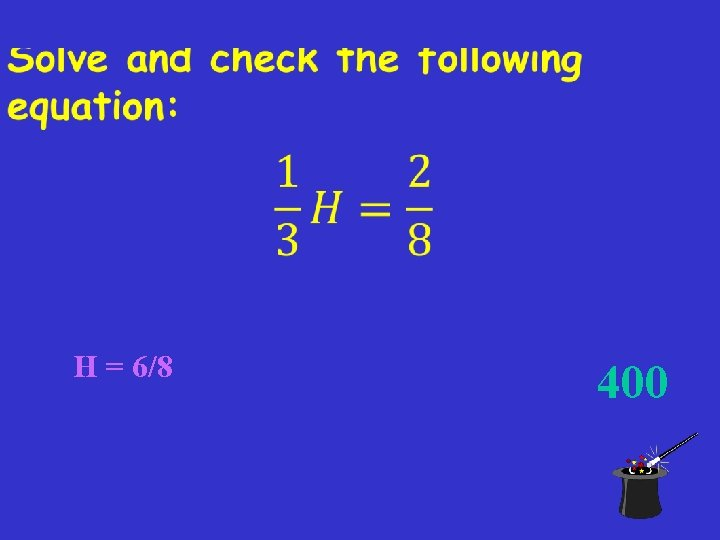H = 6/8 400T = 12 / 24 500What is the formula for the area of a rectangle? 100 A = L∙WWhat is the area of the following figure? 200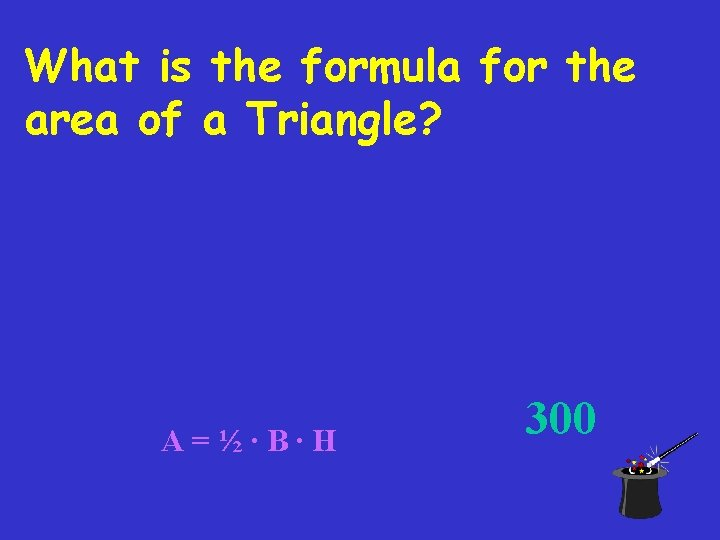What is the formula for the area of a Triangle? A=½∙B∙H 300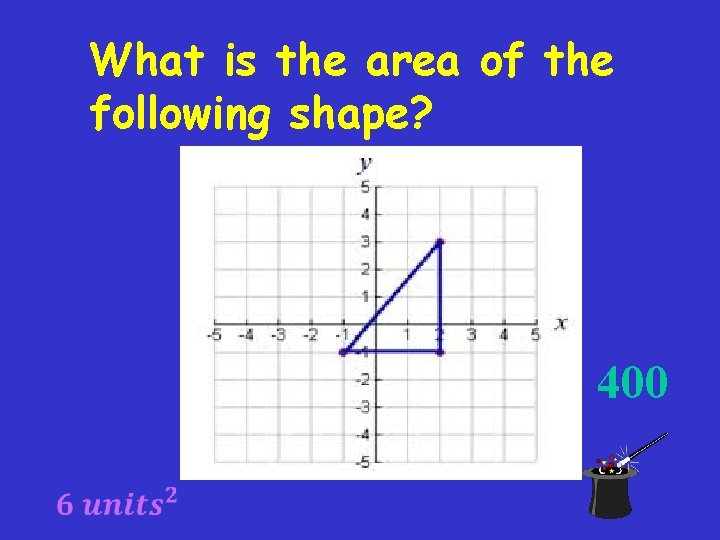What is the area of the following shape? 400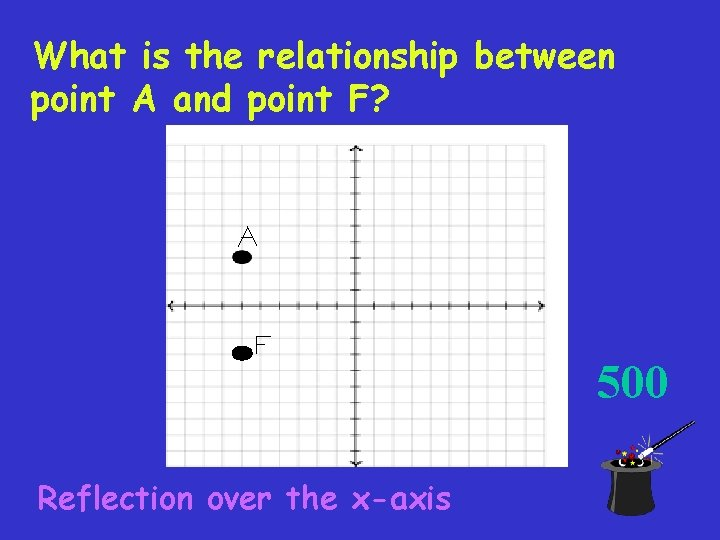What is the relationship between point A and point F? 500 Reflection over the x-axisWhat is the variable for “constant”? K 100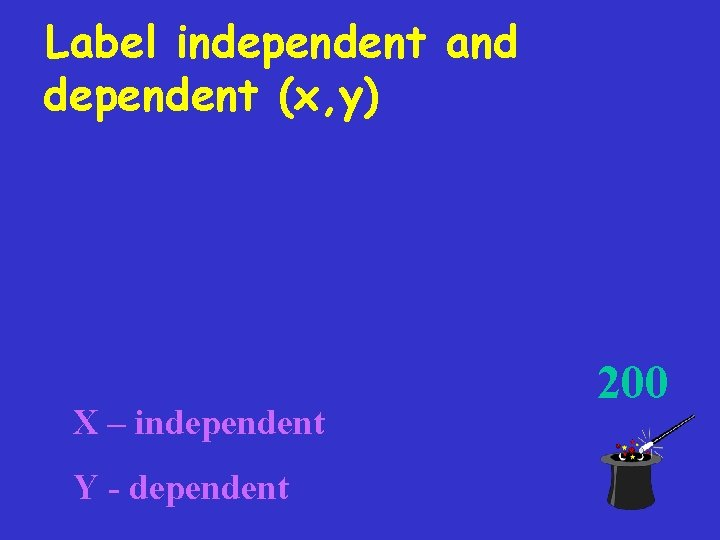Label independent and dependent (x, y) X – independent Y - dependent 200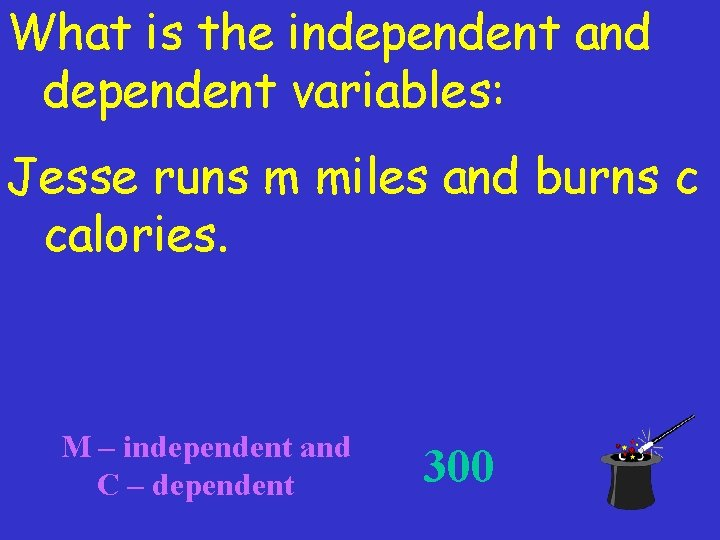What is the independent and dependent variables: Jesse runs m miles and burns c calories. M – independent and C – dependent 300The table shows the relationship between the number of pineapples bought and the total cost. What is the constant, the independent and dependent variable? K: 12. 50 Number Bought Total Cost 10 \$25 15 \$37. 50 20 \$50 30 \$75 Independent: Number bought Dependent: Cost 400The graph below represents the number of miles Michael ran over time. What is the unit rate, the independent and dependent variables? K: 5 In: Minutes D: Miles 500